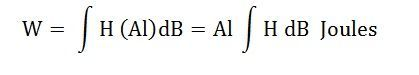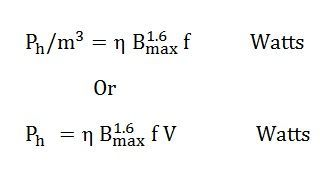# Hysteresis Loss

Definition: The work done by the magnetising force against the internal friction of the molecules of the magnet, produces heat. This energy which is wasted in the form of heat due to hysteresis is called Hysteresis Loss.

When in the magnetic material, magnetisation force is applied, the molecules of the magnetic material are aligned in one particular direction. And when this magnetic force is reversed in the opposite direction, the internal friction of the molecular magnets opposes the reversal of magnetism resulting in Magnetic Hysteresis.

To wipe out or overcome this internal friction (or in other words, known as residual magnetism), a part of the magnetising force is used. This work, done by the magnetising force produces heat; thereby causing wastage of energy in the form of heat is termed as hysteresis loss.

Let us understand this concept by taking an example of electrical machines, as the hysteresis loss occurs mainly where there is a reversal of magnetism, as in the magnetic parts of the electrical machines.

The temperature of the machine is increased as this loss results in the waste of energy, in the form of heat which is an undesirable process for the machines.

Therefore, a suitable magnetic material having a minimum hysteresis loss which has the narrow hysteresis loop is used for making these electrical machinery parts.

Magnitude of Hysteresis Loss

The figure below shows one cycle of magnetisation of the magnetic material.Consider a strip of small thickness dB on the hysteresis loop as shown in the above

For any value of current I, the corresponding value of flux is,For the small charge dϕ that are dB x A, the work done will be given as

dW = (ampere-turn) x (change of flux)The total work done during a complete cycle of magnetisation is obtained by integrating both the side of the above equation 1Where ʃ HdB is the area of the hysteresis loop

Therefore, W=Al x (area of the hysteresis loop) or

Work done /unit volume (W/m3) = area of the hysteresis loop in Joules.

Now if f is the number of cycles of magnetisation made per second, then Hysteresis loss/m3 = (area of one hysteresis loop) x (f joules/second or Watts)

Hysteresis Loss in the magnetic material per unit volume is expressed asWhere,

Ph – hysteresis loss in watts

Ƞ – hysteresis or Steinmetz’s constant in J/m3, its value depends upon the nature of the magnetic material.

Bmax – maximum value of the flux density in the magnetic material in wb/m2

f – number of cycles of magnetisation made per second

V- volume of the magnetic material (part in which magnetic reversal occur) in m3